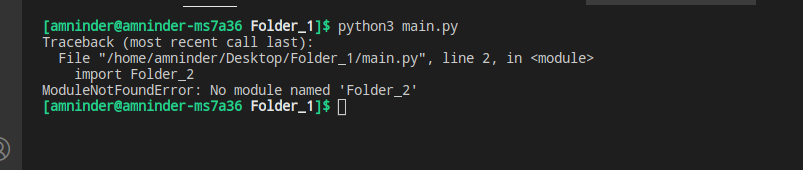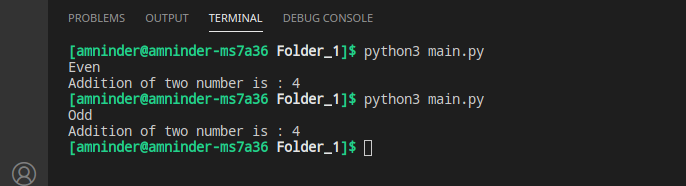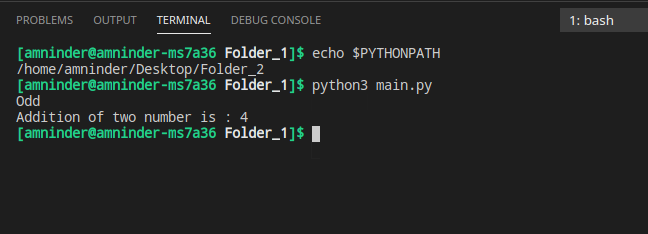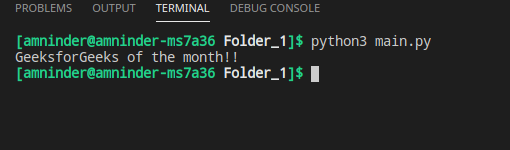Related Articles
Python – Import module from different directory
• Difficulty Level : Easy
• Last Updated : 28 Apr, 2021

While working on big projects we may confront a situation where we want to import a module from a different directory. But for some reason, the module may not be imported correctly. Now don’t worry if your module is not imported correctly. In this article, we will discuss ways to import a module from another directory.

Note: A module is just a Python program that ends with .py extension and a folder that contains a module becomes a package.

### Importing Module

Let’s suppose, we have two different folders, one contains main.py which is our main Python file where we want to import module1 from Folder_2.

Directory Structure

``` - Folder_1
- main.py
- Folder_2
- module1.py```

Module1 contains two functions called add and odd_even. The function add will takes two arguments and return the addition of them. The odd_even function will take only one argument and print Even if the number is even or print Odd if the number is odd.

## Python3

 `# creating a simple add function``def` `add(a, b):``    ``return` `a``+``b`` ` `# creating a simple odd_even function``# to check if the number is odd or even``def` `odd_even(n):``    ``if` `n ``%` `2` `=``=` `0``:``        ``print``(``"Even"``)``    ``else``:``        ``print``(``"Odd"``)`

If we simply try to import module1 from Folder_2, we will be encountering the following error.

## Python3

 `# importing module1 from another folder``import` `Folder_2`` ` `# calling odd_even function``module1.odd_even(``5``)`

Output:Error

ModuleNotFoundError, because by default python interpreter will check for the file in the current directory only, and we need to set the file path manually to import the modules from another directory. We can do this using various ways. These ways are discussed below in detail.

### Using sys module

We can use sys.path to add the path of the new different folder (the folder from where we want to import the modules) to the system path so that python can also look for the module in that directory if it doesn’t found the module in its current directory. As sys.path falls under the list type class so, we can easily use the insert method to add the folder path.

## Python3

 `# importing sys``import` `sys`` ` `# adding Folder_2 to the system path``sys.path.insert(``0``, ``'/home/amninder/Desktop/Folder_2'``)`` ` `# importing the add and odd_even ``# function``from` `module1 ``import` `odd_even, add`` ` `# calling odd_even function``odd_even(``5``)`` ` `# calling add function``print``(``"Addition of two number is :"``, add(``2``, ``2``))`

Output:Using sys

### Using PYTHONPATHenvironment variable

Similarly, if you don’t want to use the sys module to set the path of the new directory. You can assign a directory path to the PYTHONPATH variable and still get your program working.

In Linux, we can use the following command in the terminal to set the path:

export PYTHONPATH=’path/to/directory’

In Windows system :

SET PYTHONPATH=”path/to/directory”

To see if PYTHONPATH variable holds the path of the new folder, we can use the following command:

echo \$PYTHONPATH

## Python3

 `# importing the add and odd_even function``from` `module1 ``import` `odd_even, add`` ` `# calling odd_even function``odd_even(``5``)`` ` `# calling add function``print``(``"Addition of two number is :"``, add(``2``, ``2``))`

Output:Using PYTHONPATH

Suppose we have a directory structure like this:

```- project
- Folder_1
- main.py
- Folder_2
- subfolder
- new.py```

Now, you want to import the new.py module from Folder_2 to our project’s Folder_1 main.py file.

Syntax:

from project.folder.subfolder.filename import functionname

## Python3

 `# importing sys``import` `sys`` ` `# adding Folder_2/subfolder to the system path``sys.path.insert(``0``, ``'/home/amninder/Desktop/project/Folder_2/subfolder'``)`` ` `# importing the hello``from` `new ``import` `hello`` ` `# calling hello function``hello()`

Output:Output

Attention geek! Strengthen your foundations with the Python Programming Foundation Course and learn the basics.

To begin with, your interview preparations Enhance your Data Structures concepts with the Python DS Course. And to begin with your Machine Learning Journey, join the Machine Learning – Basic Level Course

My Personal Notes arrow_drop_up